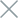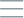• Home
• Tags
• Series
•# Greedy Search Algorithm in C++ - Explanation, Example and Code

The Greedy Search algorithm is a problem-solving approach that always chooses the best available option at each step without considering the long-term impact of the decision. While it doesn't guarantee finding the globally optimal solution, this method often works quickly and is straightforward to implement.

## How It Works

2. Local Optimal Choice: At each step, choose the locally optimal choice based on the objective function or defined criteria.
3. Apply Choice: Apply the optimal choice to the current solution.
4. Repeat: Iterate through steps 2 to 4 until no better local choice can be made.

## Example: Knapsack Problem

Consider the Knapsack Problem, where we have a knapsack with a maximum weight and a list of items with weights and values. The goal is to select items to maximize the total value in the knapsack. A Greedy Search approach for this problem is to select items based on the highest value-to-weight ratio.

## Code Example in C++

``````#include <iostream>
#include <vector>
#include <algorithm>

struct Item {
int weight;
int value;
};

bool compare(Item a, Item b) {
double ratioA = (double)a.value / a.weight;
double ratioB = (double)b.value / b.weight;
return ratioA > ratioB;
}

double greedyKnapsack(int maxWeight, std::vector<Item>& items) {
double totalValue = 0.0;

std::sort(items.begin(), items.end(), compare);

for (const Item& item : items) {
if (maxWeight >= item.weight) {
totalValue += item.value;
maxWeight -= item.weight;
} else {
totalValue += (double)maxWeight / item.weight * item.value;
break;
}
}

}

int main() {
int maxWeight = 10;
std::vector<Item> items = {{2, 6}, {5, 12}, {3, 8}, {7, 14}, {4, 10}};
double maxValue = greedyKnapsack(maxWeight, items);

std::cout << "Max value in knapsack: " << maxValue << std::endl;

return 0;
}
``````

In this example, we use the Greedy Search approach to solve the Knapsack Problem. We sort the items based on the descending value-to-weight ratio and select items with the highest ratio that still fit within the knapsack's weight limit.